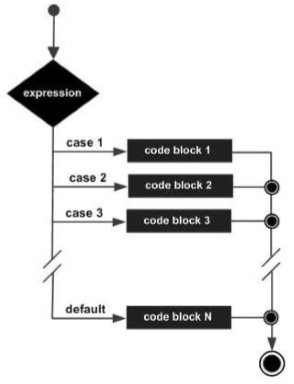# Groovy - Switch Statement

Sometimes the nested if-else statement is so common and is used so often that an easier statement was designed called the switch statement.

```switch(expression) {
case expression #1:
statement #1
...
case expression #2:
statement #2
...
case expression #N:
statement #N
...
default:
statement #Default
...
}
```

The general working of this statement is as follows −

• The expression to be evaluated is placed in the switch statement.

• There will be multiple case expressions defined to decide which set of statements should be executed based on the evaluation of the expression.

• A break statement is added to each case section of statements at the end. This is to ensure that the loop is exited as soon as the relevant set of statements gets executed.

• There is also a default case statement which gets executed if none of the prior case expressions evaluate to true.

The following diagram shows the flow of the switch-case statement.Following is an example of the switch statement −

```class Example {
static void main(String[] args) {
//initializing a local variable
int a = 2

//Evaluating the expression value
switch(a) {
//There is case statement defined for 4 cases
// Each case statement section has a break condition to exit the loop

case 1:
println("The value of a is One");
break;
case 2:
println("The value of a is Two");
break;
case 3:
println("The value of a is Three");
break;
case 4:
println("The value of a is Four");
break;
default:
println("The value is unknown");
break;
}
}
}
```

In the above example, we are first initializing a variable to a value of 2. We then have a switch statement which evaluates the value of the variable a. Based on the value of the variable it will execute the relevant case set of statements. The output of the above code would be −

```The value of a is Two
```
groovy_decision_making.htm